Get instant live expert help with Excel or Google Sheets“My Excelchat expert helped me in less than 20 minutes, saving me what would have been 5 hours of work!”

#### Post your problem and you’ll get Expert help in seconds.

Your message must be at least 40 characters
Our professional Expert are available now. Your privacy is guaranteed.

# VLOOKUP vs. Index Match: Which Is More Efficient?

The VLOOKUP function is very powerful. However, it has some restrictions which is why INDEX MATCH is the game changer. This tutorial will walk through the VLOOKUP restrictions and why INDEX MATCH is a better solution

## Final Formula

`=INDEX(A3:B8,MATCH(E2,B3:B8,0),1)`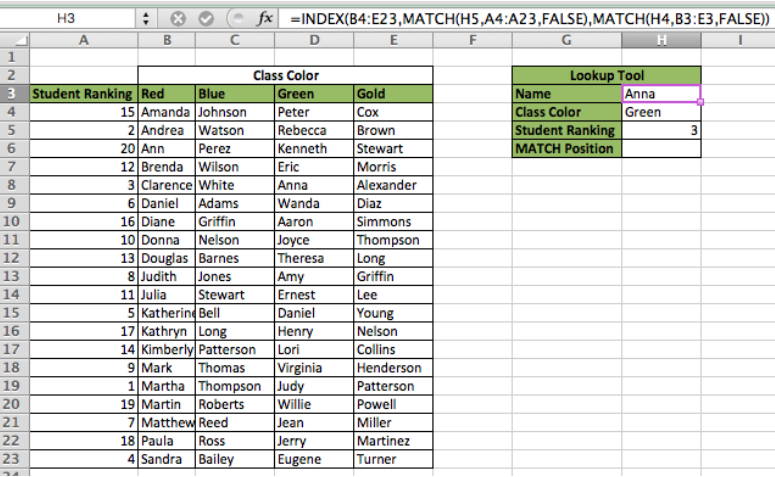Figure 1 – Final result

The VLOOKUP function has three main limitations that can be easily removed by replacing VLOOKUP with INDEX MATCH

1. The Lookup Array must be the leftmost data in the Table Array
2. The Lookup Array must be in ascending order
3. The lookup request must be only one dimensional

Now we will walk through an example of each of the three restrictions and then explain how INDEX MATCH can be applied to solve them, but let’s first setup our data

## Setting up our Data

We will setup our data so that Column A will be Student Ranking (1- 20), Columns B:E will be the Student Names. Notice in Row 3 how we added a class color. This will be used later to solve a multi-dimensional lookup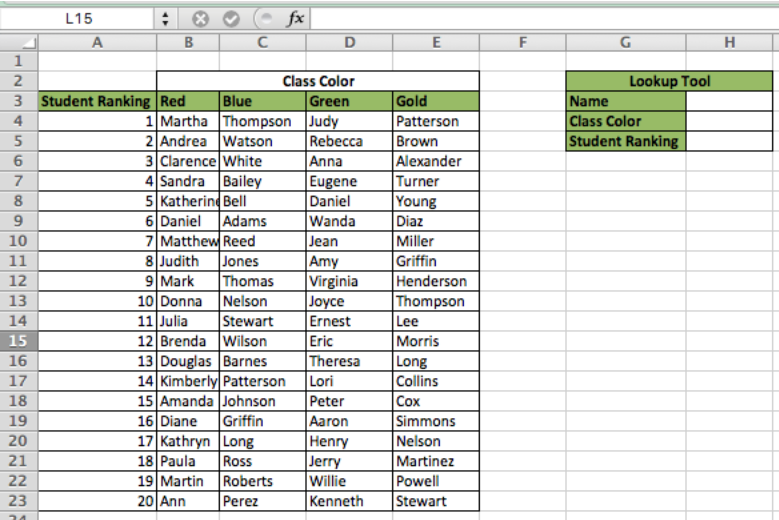Figure 2 – Setting up our Data

## VLOOKUP Restriction Number 1

As the data is currently setup now, we could never use a name in columns B:E to lookup their ranking due to the fact that VLOOKUP functions cannot return a value left of the Lookup Array. We will now work through an example:

VLOOKUP Syntax: VLOOKUP(Lookup Value, Table Array, Return Column, Approximate Match [TRUE/FALSE])

• In cell H3, type “Sandra”
• In cell H5, type this formula `=VLOOKUP(H3,A4:E23,1,FALSE)`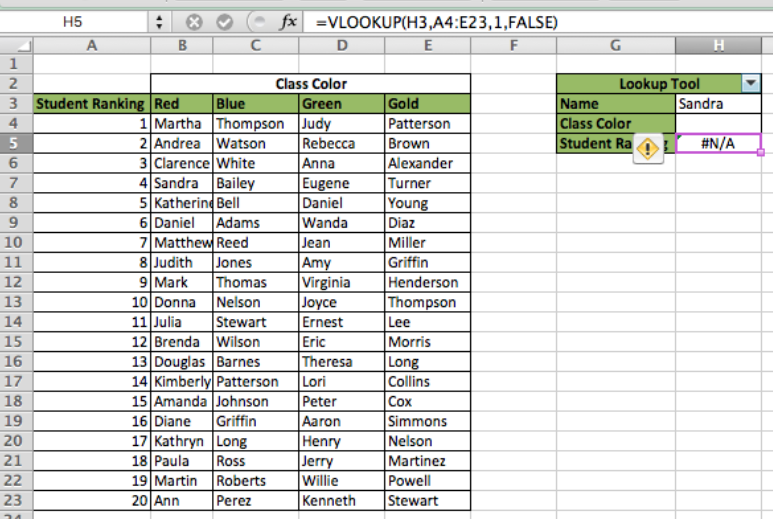Figure 3 – VLOOKUP Leftmost Error

We notice in this Example that our formula returned an #N/A error as we expected. Soon, we will work through correcting the error by replacing VLOOKUP with INDEX MATCH, but first, we must cover a key difference with INDEX MATCH. It is a super function with two different functions nested together – INDEX function and MATCH function

## Explaining the INDEX Function

The INDEX Function can return a value by taking looking at a table array and then looking at the specified row and column of the Table Array.

INDEX Syntax: `=INDEX(Table Array, Row Position, Column Position)`

• First, delete all information in cells H3:H5
• Enter this formula in H3 `=INDEX(A4:E23,3,3)`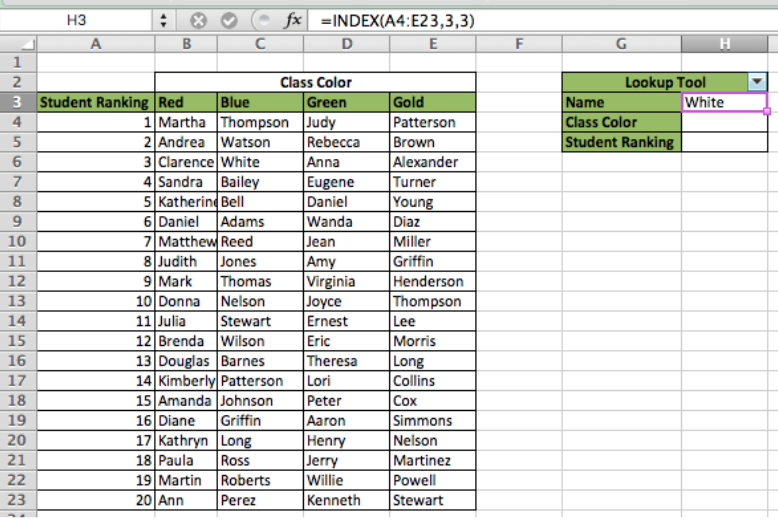Figure 4 – INDEX

We notice in this example the formula returns “White” since that is the value located in row 3 and column 3 of the Table Array. Next we will cover the MATCH function

## Explaining the MATCH Function

The MATCH Function will return the position (Row or Column) of where a Match to the Lookup Value is found

MATCH Syntax: `=MATCH(Lookup Value, Lookup Array, Approximate Match [TRUE/FALSE])`

• In cell G6, add a new category titled “MATCH Position”
• In cell H6, enter this formula `=MATCH(H3,C4:C23,FALSE)`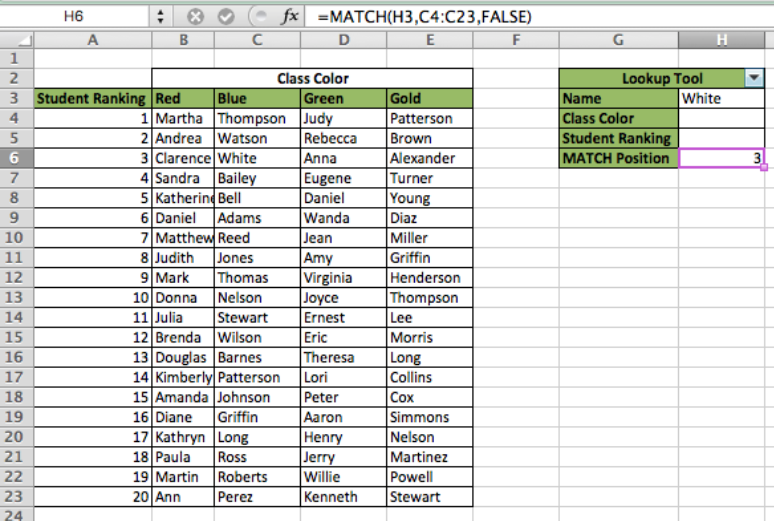Figure 5 – MATCH

We can see in this Example how the formula returns “3” because that is the row position of the matching value which is “White” in this example.

## Nesting the INDEX and MATCH Functions

When we nest the INDEX and MATCH functions, the INDEX function works the same as above. It looks at a specified Table Array for the data, except for now, the MATCH function will specify the Row Position versus it being typed in

INDEX MATCH Syntax: `=Index(Table Array, MATCH(Lookup Value, Lookup Array, Approximate Match [TRUE/FALSE])`

• In cell H5, type this formula `=INDEX(A4:A23,MATCH(H3,C4:C23,FALSE))`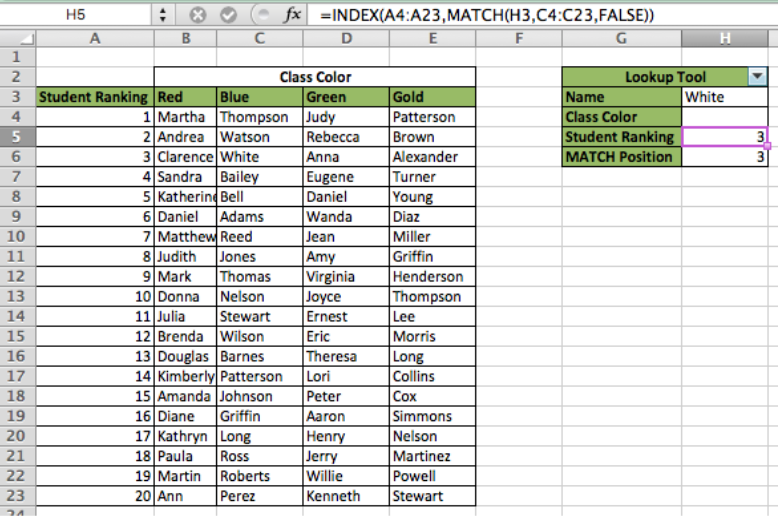Figure 6 – INDEX MATCH Explanation

We can see in this example that the formula returned a Student Ranking of “3” because “White” is positioned in Row 3 of the Lookup Array Now that we know how the MATCH function works, we can solve our VLOOKUP Leftmost restriction

## INDEX and MATCH Leftmost Solution

Since the INDEX function has the ability to specify a Row and Column, we can tell the function where we want it to look – even to the left of our Lookup Value! To illustrate, we will attempt the same VLOOKUP as before (finding Student Ranking using Name)

• First, delete all information in cells H3:H6
• In cell H3, type “Sandra”
• In cell H5, type this formula `=INDEX(A4:A23,MATCH(H3,B4:B23,FALSE))`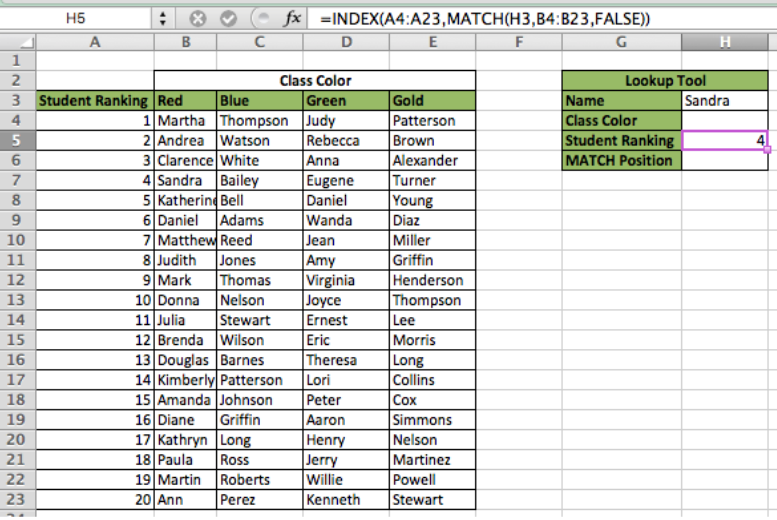Figure 7 – INDEX MATCH Leftmost

We see in this example, the formula returns a Ranking of “4” since that is the Row position where the match to “Sandra” is found. Next, we will look at the Ascending Order restriction of VLOOKUP

## VLOOKUP Restriction Number 2

We will use the same dataset, but to illustrate this point, we will unsort the Student Ranking Column. To do this, we will sort the data by Column B. See the figure below: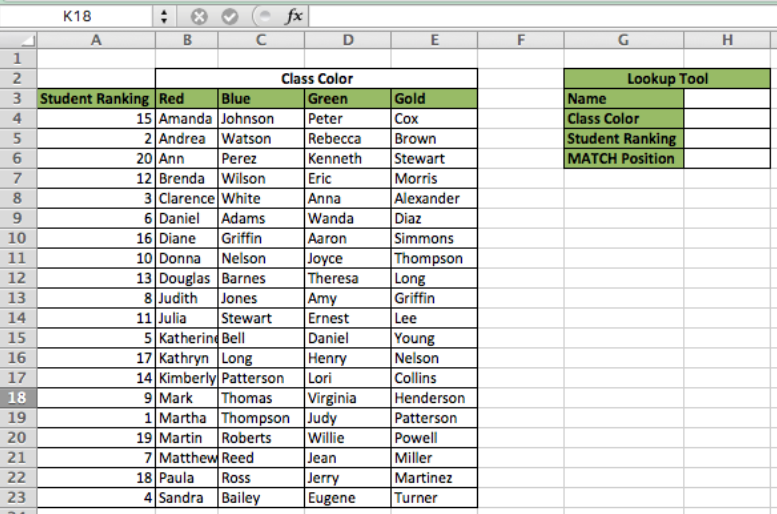Figure 8 – Data Table Re-sorted

Now that we have the data sorted out of order, we will attempt to return the Student associated with the Lookup Value (Student Ranking). First we will attempt with VLOOKUP

• First, delete all information in cells H3:H6
• In cell H5, type “3”
• In cell H5, type this formula `=VLOOKUP(H5,A4:E23,2,FALSE)`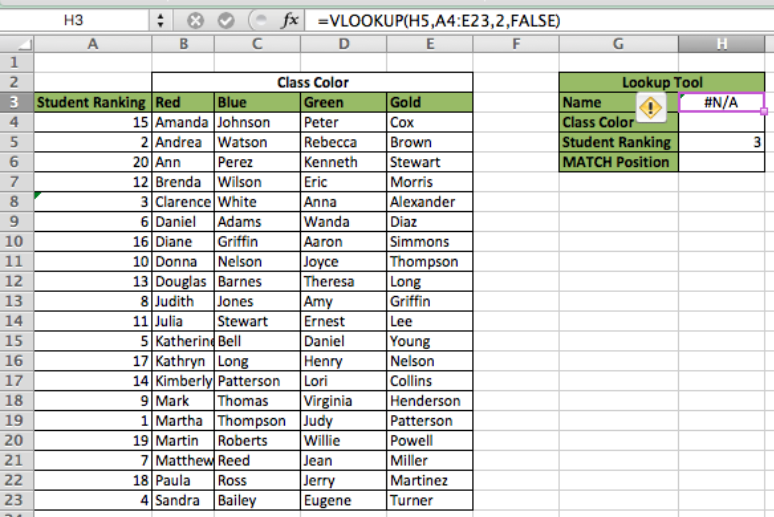Figure 9 – VLOOKUP Unsorted

As we can see from this example, the formula returns an error because the data is not sorted properly. Another potential issue that could occur is that the lookup returns the wrong value which could be a very costly if not caught. Now we will solve this using INDEX MATCH

• INDEX MATCH Ascending Order Solution
• In cell H5, type this formula `=INDEX(B4:B23,MATCH(H5,A4:A23,FALSE))`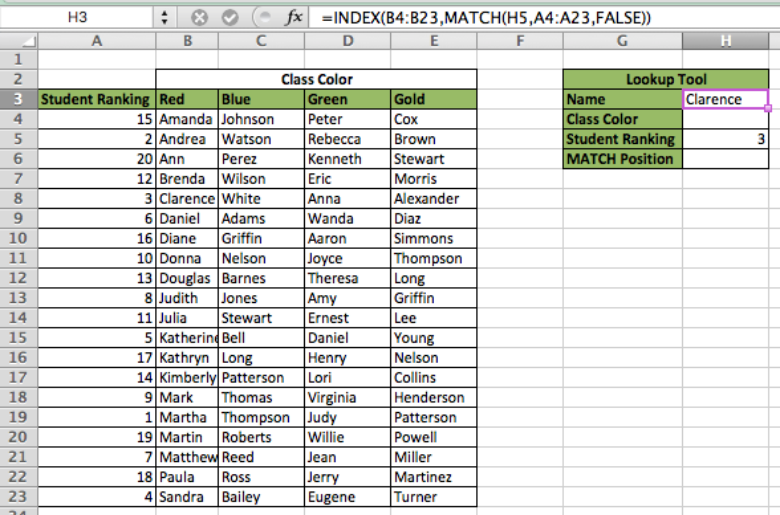Figure 10 – INDEX MATCH Asscending Order Solution

In this Example, we see how the formula returns “Clarence” and solves the Asscending Order restriction. Now we will explain the main reason INDEX MATCH is a game changing formula

## VLOOKUP Restriction Number 3

The VLOOKUP formula is limited to one lookup column. Therefore, we are unable to lookup a Column and a Row in order to create a dynamic lookup. Consider an example where we want to know the name of the student with a ranking of “3” in the “Green” class. This is impossible using VLOOKUP.

## INDEX MATCH Multi-Dimensional Solution

• First, delete all information in cells H3:H6
• In cell H4, type “Green
• In cell H5, type “3”
• In cell H3, type this formula `=INDEX(B4:E23,MATCH(H5,A4:A23,FALSE),MATCH(H4,B3:E3,FALSE))`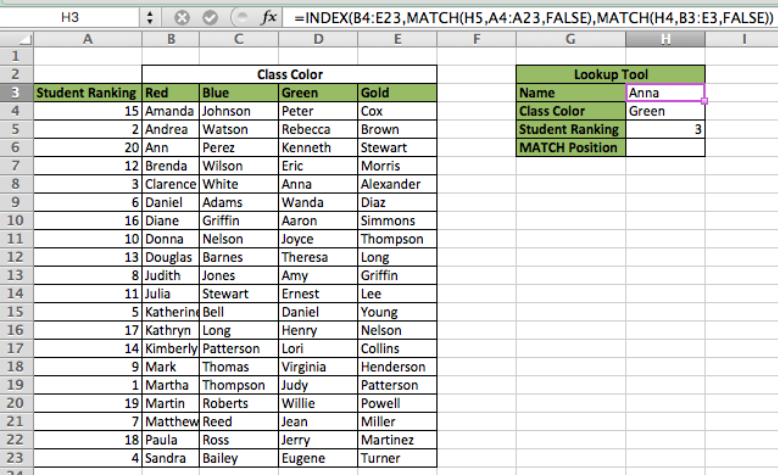Figure 11 – INDEX MATCH Multi-Dimensional Solution

We can see in this example that the formula returns “Anna” because the matching row for Student Ranking of “3” is 3 and the matching Column position for “Green” is 3. Therefore, if we look for the Index of Row 3, Column 3 in the data table, we will get the result of Anna. With this capability, the INDEX MATCH function is one of the most powerful and dynamic functions in Excel

## Instant Connection to an Expert through our Excelchat Service

Most of the time, the problem you will need to solve will be more complex than a simple application of a formula or function. If you want to save hours of research and frustration, try our live Excelchat service! Our Excel Experts are available 24/7 to answer any Excel question you may have. We guarantee a connection within 30 seconds and a customized solution within 20 minutes.

### Did this post not answer your question? Get a solution from connecting with the expert.Another blog reader asked this question today on Excelchat:
Solution examplesI'm looking for a formula or format for if there is data in c4 on a sheet... It adds extra information underneath
Solved by X. E. in 20 minsI need a formula to compare the data in two columns and then export the mismatched data in the 3rd column
Solved by S. Q. in 20 minshow to make a format that if multiple cells have number contents its value is 1 in the last cell
Solved by C. C. in 28 minsI have a file with two sheets. On the 1st, I have dollars in O13and in O16 a number signifying 1-6 people. On the second workbook is a matrix that corresponds to the dollar amount AND the number of people. For example, sheet 1 O13 is \$6,000. O16 is 3. I want a formula to find \$6,000 (not higher) on sheet 2 in column A. Then I want it to compare sheet 1 O16 to the correct column (B-G representing 1-6) on sheet 2 for an exact match to place in sheet1 O17. The range of sheet1 O13 is referenced on sheet2 A5-A383, and sheet 1 O16 is referenced on sheet2 B5:G383. I have an INDEX Match but it isn't quite working, yet.
Solved by B. B. in 60 minsI have two lists. One is a list of part numbers. The other is a list of long text descriptions. I can use VLOOKUP with "*"&cell address"*" to find the first match. But how can I find the second and third matches? I have tried using INDEX and SHORT but the wild cards no longer find the match like they do in VLOOKUP. Can you help?
Solved by S. J. in 36 mins## Subscribe to Excelchat.coAnother blog reader asked this question today on Excelchat: